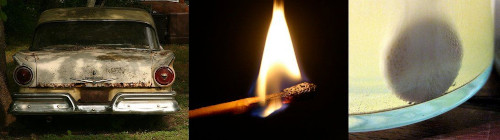We think you are located in United States. Is this correct?

# Test yourself now

High marks in science are the key to your success and future plans. Test yourself and learn more on Siyavula Practice.

# Chapter 7: Rate and Extent of Reaction

In this chapter learners will look at the rates of different reactions and the factors that affect those rates. In grade 11 learners were introduced to the energies involved in chemical reactions as well as activation energy graphs. These topics will be explored more thoroughly in this chapter. It is important that this section is covered thoroughly to give the learners a deep understanding that they can use in the following chapters (particularly Chapter 8).

The following topics are covered in this chapter.

• What the term reaction rate means

In this section learners will look at what a reaction rate is and the different ways of determining the rate of a reaction. They will do calculations based on the rates of different reactions.

• The factors affecting reaction rates

Learners will be introduced to the concept of collision theory as a way of understanding why certain factors affect the rate of a reaction. They will investigate (with experiments) how the nature of the reactants, the surface area, the concentration and the temperature affect the rate of a reaction. The effect of a catalyst will also be studied.

• How to measure rates of reaction

In this section learners will look at methods of measuring reaction rates including the collection of gas, the formation of precipitate, a change in colour and a change in mass. They will perform experiments and calculate reaction rates to better understand these concepts.

• The mechanisms of reaction and the effect of catalysts

In this section learners will explore the mechanisms behind reaction rates, specifically focusing on activation energy. The effect of catalysts on activation energy will be covered in detail.

There are several experiments in this chapter. The learners will be using dangerous chemicals and should be properly instructed on the correct use of safety equipment, including safety goggles, gloves and protective clothing. They should also be reminded not to sniff any chemicals as the fumes can be dangerous as well. More information on laboratory procedures as well as safety precautions is provided in Chapter 1 (Science skills).

In the informal assessment the learners must determine a quantitative reaction rate in the reaction of $$\text{Na}_{2}\text{S}_{2}\text{O}_{3}$$ and $$\text{HCl}$$. Learners are required to perform the experiment, calculate quantitative reaction rates (using the formation of precipitate), and plot a graph of their results.

## 7.1 Introduction (ESCMW)

A car slowly rusting away, a match burning, a tablet dissolving in water. All of these are chemical reactions and all of these take place at different rates. If you were to hold a race between these three reactions you would find that the match wins (it burns quickly), while the car rusting would lose (it may take years to rust).Figure 7.1: A rusting car, a match burning and a tablet dissolving in water.

In this chapter we will look at why reactions proceed at different rates (speeds) and how we can change the rate of the reaction.

In physics, speed is the scalar measurement that tells us how fast or slow an object is moving (position vs. time). For reactions the speed (or rate) of a reaction means how fast or slow the reaction is and indicates the amount of product formed and the amount of reactants used per unit time.